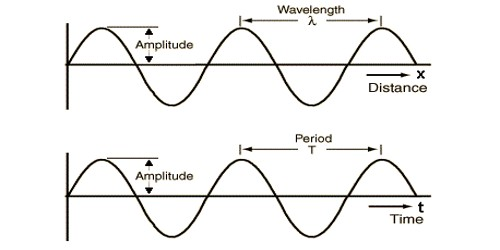# Explain the Relation between Frequency and Time Period

We know that the number of complete vibrations of a vibrating particle in one second is called its frequency. Frequency is denoted by f. Again the time period is the time of one complete vibration. Frequency and period are inversely related quantities. The frequency (f) of a wave is the number of full waveforms generated per second. This is the same as the number of repetitions per second or the number of oscillations per second.

Time Period (T) is the number of seconds per waveform or the number of seconds per oscillation. It is clear that the frequency and time period are reciprocals.

If the time period is T, then in T second, the number of vibration is 1

Therefore, the number of vibration in one second is = 1/T

The number of vibration in 1 second is frequency. So, the frequency, f = 1/T

Frequency refers to how often something happens; whereas Period refers to the time it takes for something to happen. Frequency means how many times a periodic event happens in a second.
A period is a time between any 2 events. As a general rule, the period should be the reciprocal of the frequency, hence the equation: Period = 1/Frecuency.

Frequency, f, is how many cycles of an oscillation occur per second and is measured in cycles per second or hertz (Hz). The period of a wave, T, is the amount of time it takes a wave to vibrate one full cycle. These two terms are inversely proportional to each other: f = 1/T, and T = 1/f.For example, if a wave takes 1 second to oscillate up and down, the period of the wave is 1 second. The frequency is the reciprocal of that, 1 cycle/sec because only one cycle occurred in a second.

Mathematical example: The wavelength of sound produced by an object in the air is 20 cm. If the velocity of sound in the air is 340 ms-1, find the frequency and period of the object.

Here, Wave-length, γ = 20 cm = 0.2 m

Sound-velocity = 340 ms-1

Frequency, f =?

Period (time), T = ?

We know, Velocity = fγ

So, f = v/γ = 340 ms-1 / 0.20 m = 1700 Hz

And T = 1/f = 1 / 1700 s-1 = 0.000588 s = 5.88 x 10-4 s

The frequency 1700 Hz; Period (time) 5.88 x 10-4 s (Inverse because if the frequency is high, then the time period is low).

Frequency is a rate quantity. The period is a time quantity. Frequency is the number of cycles/second denoted as Hertz (Hz). The period is the seconds/cycle. Period – in time – is measured in seconds, hours, days or years.